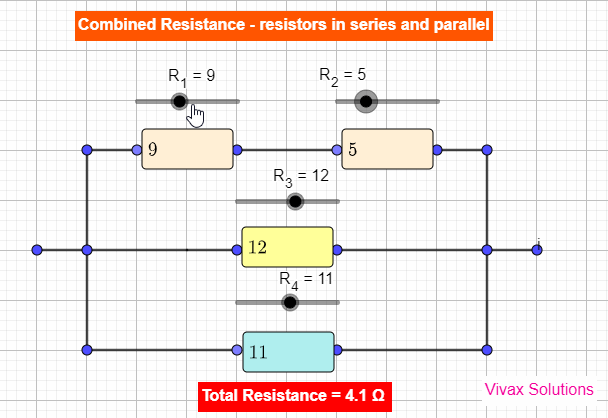### Total resistance of combined resistors - series and parallel circuitsResistors in series and parallel

Resistors can be combined in two different methods; they are in series and in parallel.
If two resistors, R1 and R22, are in series, the total resistance, Rt, is given by the following formula.
Rt = R1 + R2
If the same resistors are in parallel, the total resistance is as follows:
Rt = 1/R1 + 1/R2

E.g. 1
Two resistors, 6 and 3 are connected in series. Find the total resistance.
Rt = R1 + R2
Rt = 6 + 3
Rt = 9

E.g.2
Two resistors, 10 and 15 are in parallel. Find the total resistance.
1/Rt = 1/10 + 1/15
1/Rt = 5/30
Rt = 6

If resistors are in series
• The total resistance is bigger than the highest individual resistance of the circuit.
• The current through each resistor is the same.
• The total voltage splits up across each resistor.
• If one resistors is removed, the currents does not flow through the entire circuit
E.g. Christmas tree decorating lights

If resistors are in parallel

• The total resistance is smaller than the highest individual resistance of the circuit.

• The voltage across each resistor is the same.
• The total current splits up across each resistor
• The loss of a resistor does not affect the functioning of the circuit
E.g. The domestic electric circuits

You can practise the resistors in series and parallel with the following applet:

For a more comprehensive tutorial with lots of worked examples on electricity, please use the following link on the main site:
Electricity tutorial